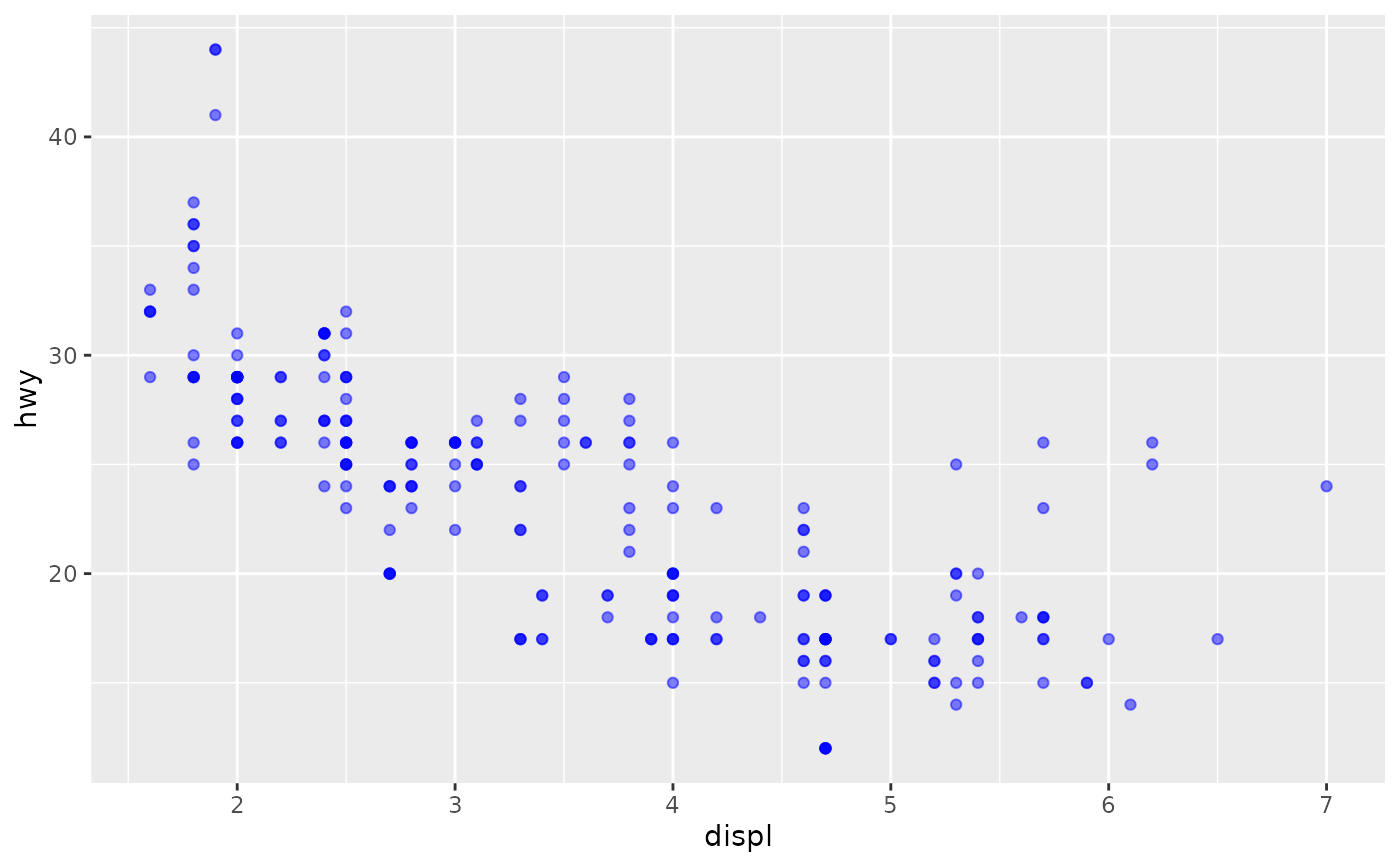These objects are imported from other packages. Follow the links below to see their documentation.

grid

arrow, unit

scales

alpha

## Examples

ggplot(mpg, aes(displ, hwy)) +
geom_point(alpha = 0.5, colour = "blue")ggplot(mpg, aes(displ, hwy)) +
geom_point(colour = alpha("blue", 0.5))